|

# 北欧风、日式风才算正确?被妖魔化的「轻中式」不服!

不知道从什么时候开始，讲到风格首推北欧风、日式风。我记得之前看过一篇文章，标题是这样的：『为什么中国人装不好北欧风？』我摔~废话，因为我们是中国人啊！为什么一定要装好北欧风，我就喜欢浓艳KTV风格了，你管得着吗？

当然这两个风格没毛病，我自己也很喜欢。但仔细想想，总觉得有点儿不对劲，后来我想明白了，有毛病的大部分时候都是人。

进入家具行业也有一段时间了，也发现要让身边国人的审美提升需要一定的时间，毕竟格调这东西都是从土里面慢慢长出来的，但不代表我们就要对新中式充耳不闻，甚至是粗暴地妖魔化。总是看到有人说新中式家具全是符号堆砌，是对明清家具外形上进行修修补补，没有的独立理念。

中式虽好，就是太老？

提到新中式，你的脑海里可能会马上出现这种僵硬的七件套，一成不变的万字纹，高大的土豪吊顶，那么，新中式真是这样的吗？既然要与现代融合，怎么可能不加入北欧MUJI等等的先进设计呢？

下面这张就是新中式的「整体风格」，我相信你一定见过更丑的。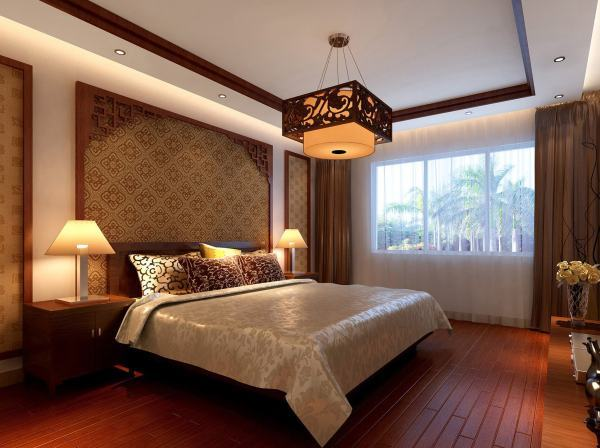与之相伴的就是我们今天要谈的「设计玄学」，新中式一定要有「整体风格」，而「整体风格」就意味着：不能按我们的个性选择点缀，不能随我们审美的进步而演进，僵化呆板，没有活力，有一种用力过猛的沉闷感。

有人会说，没有整体风格，就很容易不伦不类啊！的确，有一些「混搭」设计是有说不出来的别扭。来个大家看看「新中式」的高阶段位洗洗眼睛，法云安缦酒店：当然这是精品酒店，看着就特别贵，美虽美，却不接地气，平常人家想装修弄成这样？那你得先给我那么大一间房。但是你要说完全没有借鉴意义吗？且看下文。

现在提到的“新中式”，我更愿意理解为“融入现代东方元素家具”或者叫“现代中式”，既然如此，从某种程度上来说它和目前时下流行的北欧风、日式风都是可以结合的。

从被玩坏了的「新中式」里出逃，我们这次来讲讲更适合年轻人的「轻中式」。

1.与北欧风结合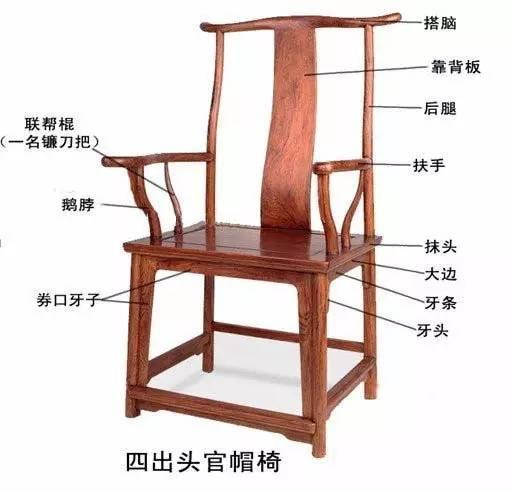中式太师椅确实稍显僵硬，它的设计就是为了让人「正襟危坐」，当然官帽椅靠背其实也有「S曲线」啦。只不过当时人体工学不够发达，稍后说说演变更多的北欧设计。

新中式和北欧风的搭配，最大的坑就是「廉价」，就是用廉价的材料和设计替代中式的「质感」，这样既失去了中式的古韵，也得不到北欧的舒适。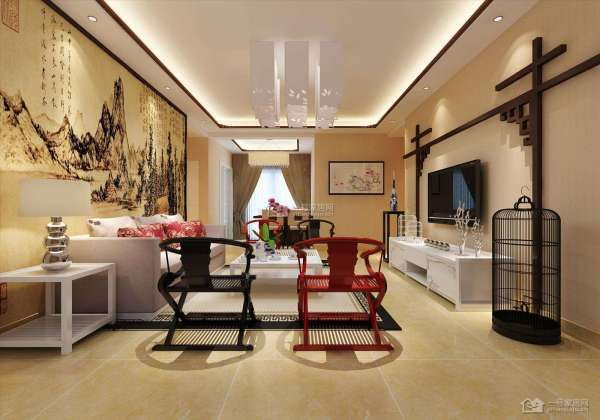看到这幅图的时候，第一反应是，是有多么热爱「红」木家具？不说电视墙上看不出所以然的形制花纹，那个鸟笼和宜家同款边几又是什么鬼？另外你数数图上有几种颜色？说说配色，虽然北欧以「高饱和度色」著称但「中国红」 的饱和度要低一些才有「古韵」 ，并不是一定要用昂贵的红木家具。

新中式如何得当的配色？我们可以去国画中找找灵感，不要再谈红色变，一样可以用的很高级：清冷寡欲版：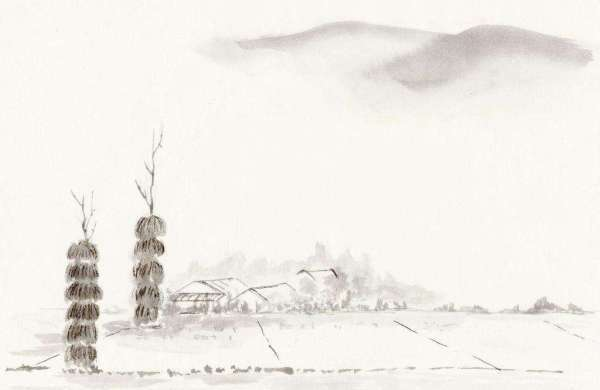下面讲讲元素方面的融合。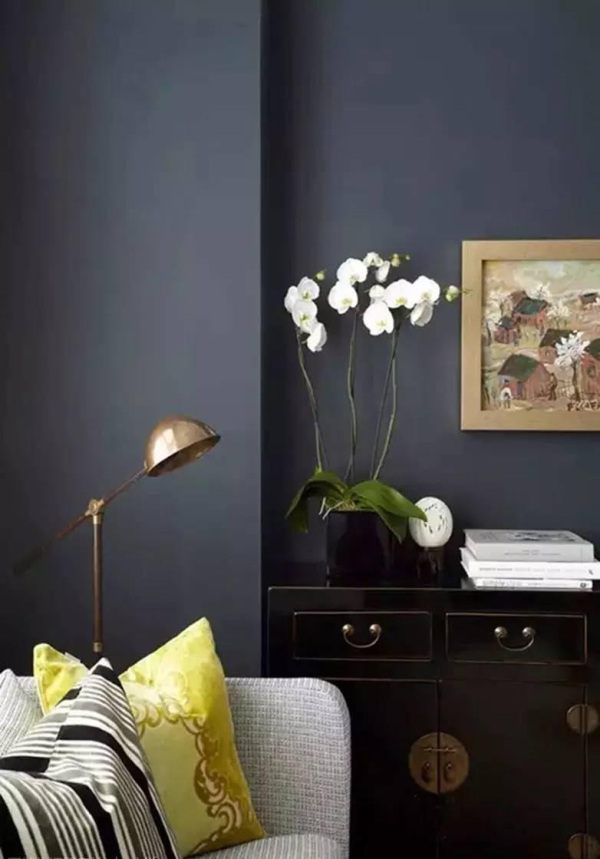上图的白色沙发就有不俗的质感，此外中式家具本就有「包铜包铁」的传统，正好融合北欧的「黄铜」落地灯。混搭古典与现代元素的专家Benjamin Dhong，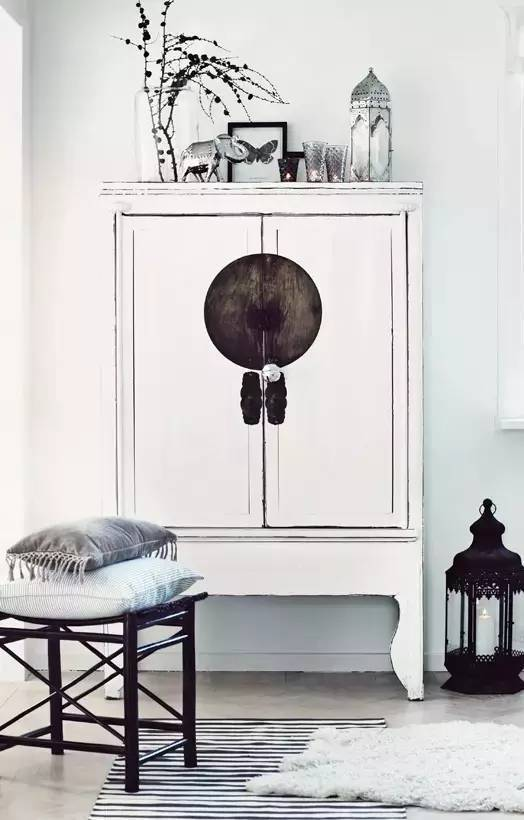巧妙将成白色的中式案几，与北欧装饰、金属配饰和谐搭配。整个空间色彩简约自然，也符合中式意境的经典、宁静和「留白」。提到用北欧的「舒适」来调和中式的「方正」。就绕不过这张Y chair/叉骨椅。它是丹麦设计大师Hans J. Wegner，借鉴「明椅」后设计的经典作品，这把椅子让丹麦的「人文」元素首次成为现代工业的一部分。而传统的太师椅至今还是昂贵而不实用。Wegner还用明椅设计过一把「The Chair」，

后来尼克松和肯尼迪辩论还用了，堪称世界上最有名的椅子。

国内的单品与北欧的搭配，比较出彩的是现在最红的独立设计品牌「梵几」，如这款「挑担茶几」，灵感来自挑水的扁担，与宜家沙发、伊姆斯摇椅搭配并不显突兀。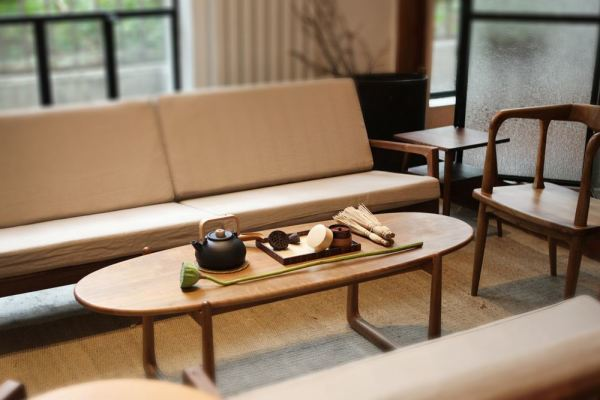梵几创始人高古奇一直就把Wegner视为典范，其设计也一直在融合北欧、日式的优良元素。

梵几另外值得一提的是传统「罗汉床」 的改进版，不但更加简约开阔，210cm的长度也可以直接卧睡。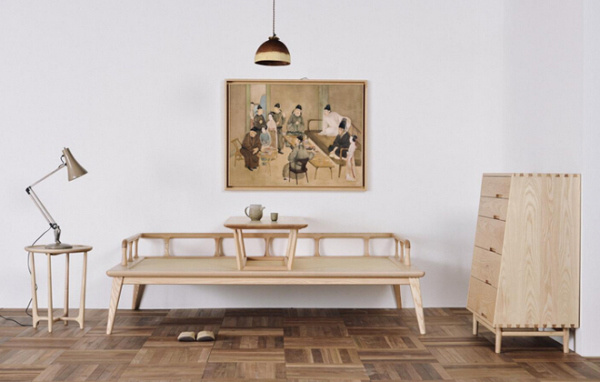梵几的缺点就是太贵了！嗯，我先把你们想说的先说了，鸡贼如我~具有轻松的色彩和柔和的形体.而「木智工坊」的「蝴蝶沙发」，则有新中式的硬朗线条，电视柜也是他们家的「山水电视柜」。

木智工坊继承的是，意大利设计大师Enzo Mari的「形式至上」，其实会更偏现代主义一些，

待会儿说。

2.与现代风结合

许多人不喜欢中式，就是因为「整体风格」，喜欢用中式元素「填满」客厅。别说我用不着红木七件套，对万字纹「审美疲劳」，光说这些款式一成不变，

而我家要与时俱进怎么办？如果说北欧与新中式是一种「调和」关系，现代主义与中式就是更高级的「冲突」或「映衬」了。而在冲突中，更能显示出主人的用心。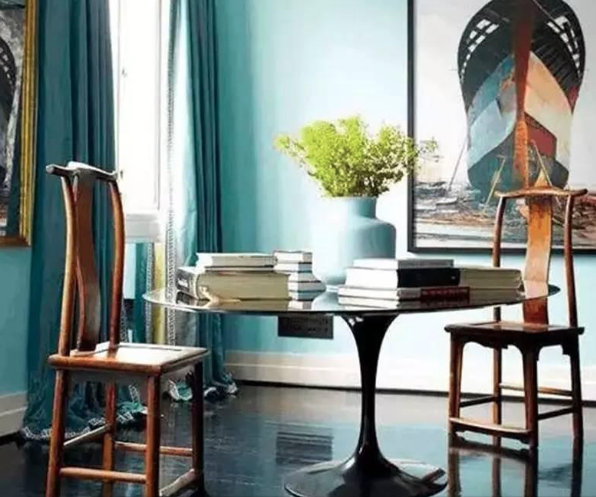上图的「官帽椅」是典型的中式元素，而「郁金香桌」是有机现代主义大师Eero Saarinen的经典作品。两相映照不仅不显得突兀，反而有一种方圆融合，现代的「张扬」与传统的「质朴」相融的感觉。另外墙壁的「婴儿蓝」与吊灯的「中国红」，也形成了巧妙的CP。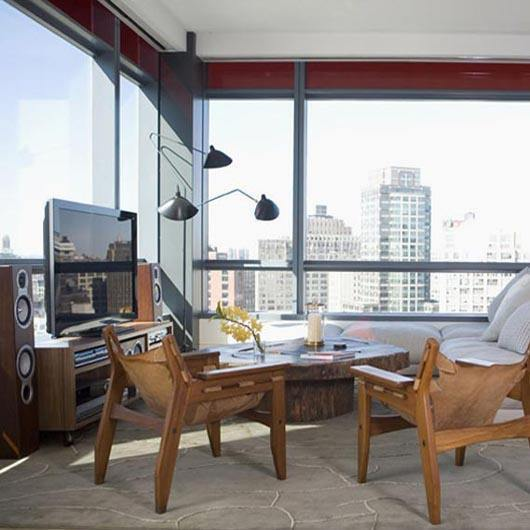如果想要更张扬，那么一定不能错过「张牙舞爪灯」。以前还介绍过同风格的落地灯。

再看看配色，现代主义的「黑白对比」清爽而有利于采光，但放在家里就有失「冰冷」，像办公室，缺乏一点「温暖」的文化韵味。加上一座中国红屏风就会「稳重浓郁」一些。如果觉得传统屏风过于厚重，可以用「失物招领」的这款新中式屏风衣架。它用最简单的线条勾勒出的屏风，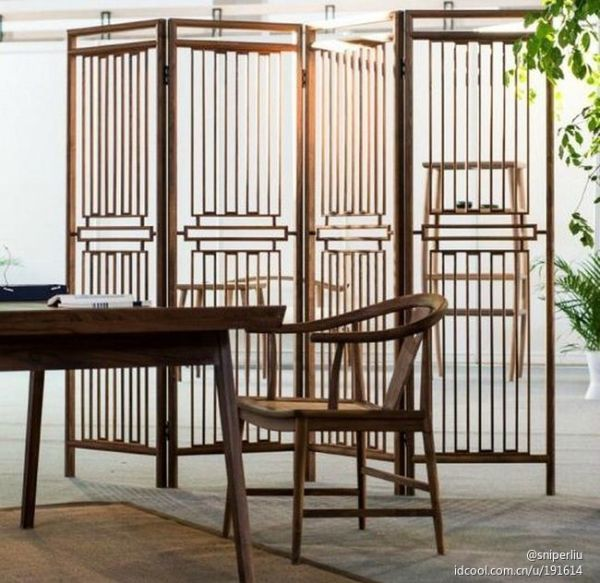可以用来划分客厅与工作室的空间，垂搭几方薄纱就能造出一片柔光。失物招领的灵感经常来自胡同口无人认领的家具，主张「珍惜」飞逝的现代进程中失去的情感。

提到现代主义，就不能不提反思日常的「厌式房间」。他家的 WAVE书架有着不俗的「流水形状」，与传统的中式风格有所不同，给极简冷淡的现代风格多加了一层灵动。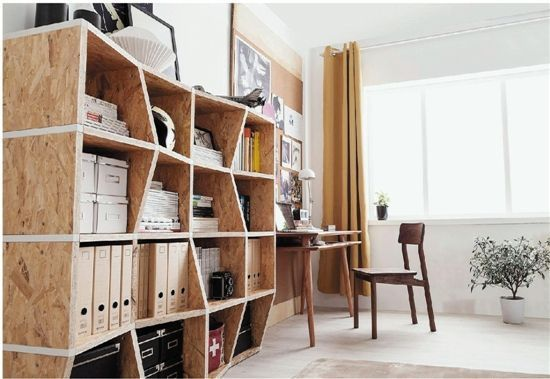或者你也可以反过来用中式的复古桌子，搭配现代的「幽灵椅」，来营造出这种冲突。注意沙发边上的「几何形花盆」、格子置物架、圆形的B&O音箱等。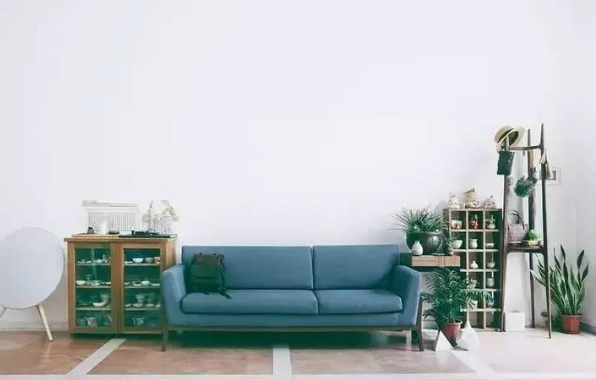梵几衣帽架的「中式山形」，与现代主义的几何形体形成强烈的对比。另外，其实传统与现代的冲突对比也有公式，这个不分东西。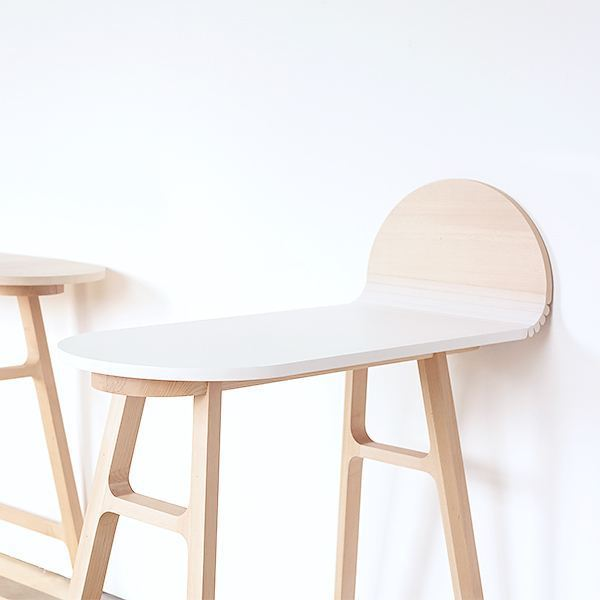自己比较喜欢「吱音」的达利吧台桌/圆桌，巧妙的融合中式的「竹简元素」与「达利艺术」，并且便于收纳，「设计是有用」的嘛！搭配现代风格更是不在话下。获2015金点奖。

再来看看这个有趣的品牌「有所设计」。这款「提梁电视柜」，灵感来源于中国古人的「提盒」，借鉴提梁处的结构元素，价格也很亲民。3.新中式不适合小户型？看看新中式+日式

相比北欧、现代风格，日式与新中式的搭配应该是最容易的。因为都以百搭的原木色为主嘛。

另外大家喜欢的汉制「琅琊榜」风格，其实比起「明清家居」，倒不如说更接近日式的「榻榻米」。去MUJI，买的可能更多是这款懒人沙发和香薰灯吧。零食也就罢了，懒人沙发总不会是从核污染区出来的吧~新中式其实有很多榻几适合榻榻米和懒人沙发，比方上图失物招领「不完全茶桌」和「不完全梳背椅」。梵几的「禅椅」，本身也带着禅意。小细腿很适合营造小户型的「留白」。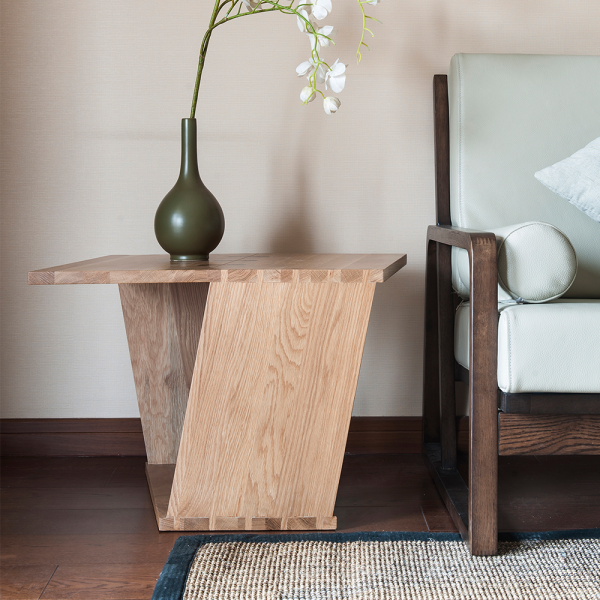朗居清源的卷几，运用几何设计美学，像折纸一样折出一张几；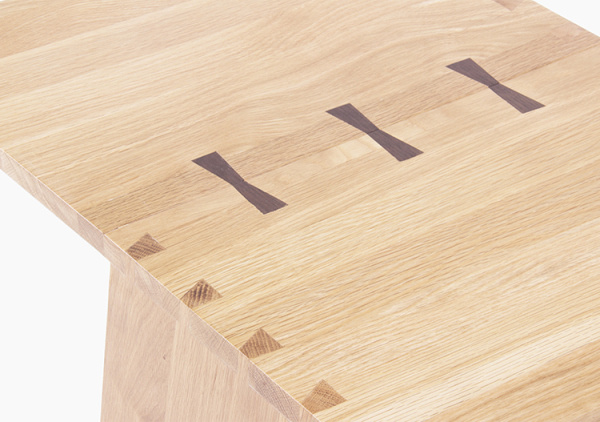用了全燕尾榫结构。

很多人提到新中式的家具显然收纳空间不足，的确存在这样的问题。所以我在这里建议大家不要全屋都用新中式家具，在素雅的空间下，取1-2件最爱作为点缀，会有让你惊喜的效果！

其实我们在讨论某种设计风格的时候，最怕的就是极左或极右：这风格一看就不实用；没有生活气息；一看就是样子货……

你们说的都对！但是从某种程度上来说，你说他造价高也好，不实惠也好，现代中式家具最吸引我的就是那股子匠人的精气神，这是工业流水线下诞生的北欧家具比不了的。

生活本来就是融合之后的样子，不是嘛？

`声明：本文由入驻焦点开放平台的作者撰写，除焦点官方账号外，观点仅代表作者本人，不代表焦点立场错误信息举报电话： 400-099-0099，邮箱：jubao@vip.sohu.com，或点此进行意见反馈，或点此进行举报投诉。`A B C D E F G H J K L M N P Q R S T W X Y Z
A - B - C - D - E
• A
• 鞍山
• 安庆
• 安阳
• 安顺
• 安康
• 澳门
• B
• 北京
• 保定
• 包头
• 巴彦淖尔
• 本溪
• 蚌埠
• 亳州
• 滨州
• 北海
• 百色
• 巴中
• 毕节
• 保山
• 宝鸡
• 白银
• 巴州
• C
• 承德
• 沧州
• 长治
• 赤峰
• 朝阳
• 长春
• 常州
• 滁州
• 池州
• 长沙
• 常德
• 郴州
• 潮州
• 崇左
• 重庆
• 成都
• 楚雄
• 昌都
• 慈溪
• 常熟
• D
• 大同
• 大连
• 丹东
• 大庆
• 东营
• 德州
• 东莞
• 德阳
• 达州
• 大理
• 德宏
• 定西
• 儋州
• 东平
• E
• 鄂尔多斯
• 鄂州
• 恩施
F - G - H - I - J
• F
• 抚顺
• 阜新
• 阜阳
• 福州
• 抚州
• 佛山
• 防城港
• G
• 赣州
• 广州
• 桂林
• 贵港
• 广元
• 广安
• 贵阳
• 固原
• H
• 邯郸
• 衡水
• 呼和浩特
• 呼伦贝尔
• 葫芦岛
• 哈尔滨
• 黑河
• 淮安
• 杭州
• 湖州
• 合肥
• 淮南
• 淮北
• 黄山
• 菏泽
• 鹤壁
• 黄石
• 黄冈
• 衡阳
• 怀化
• 惠州
• 河源
• 贺州
• 河池
• 海口
• 红河
• 汉中
• 海东
• I
• J
• 晋中
• 锦州
• 吉林
• 鸡西
• 佳木斯
• 嘉兴
• 金华
• 景德镇
• 九江
• 吉安
• 济南
• 济宁
• 焦作
• 荆门
• 荆州
• 江门
• 揭阳
• 金昌
• 酒泉
• 嘉峪关
K - L - M - N - P
• K
• 开封
• 昆明
• 昆山
• L
• 廊坊
• 临汾
• 辽阳
• 连云港
• 丽水
• 六安
• 龙岩
• 莱芜
• 临沂
• 聊城
• 洛阳
• 漯河
• 娄底
• 柳州
• 来宾
• 泸州
• 乐山
• 六盘水
• 丽江
• 临沧
• 拉萨
• 林芝
• 兰州
• 陇南
• M
• 牡丹江
• 马鞍山
• 茂名
• 梅州
• 绵阳
• 眉山
• N
• 南京
• 南通
• 宁波
• 南平
• 宁德
• 南昌
• 南阳
• 南宁
• 内江
• 南充
• P
• 盘锦
• 莆田
• 平顶山
• 濮阳
• 攀枝花
• 普洱
• 平凉
Q - R - S - T - W
• Q
• 秦皇岛
• 齐齐哈尔
• 衢州
• 泉州
• 青岛
• 清远
• 钦州
• 黔南
• 曲靖
• 庆阳
• R
• 日照
• 日喀则
• S
• 石家庄
• 沈阳
• 双鸭山
• 绥化
• 上海
• 苏州
• 宿迁
• 绍兴
• 宿州
• 三明
• 上饶
• 三门峡
• 商丘
• 十堰
• 随州
• 邵阳
• 韶关
• 深圳
• 汕头
• 汕尾
• 三亚
• 三沙
• 遂宁
• 山南
• 商洛
• 石嘴山
• T
• 天津
• 唐山
• 太原
• 通辽
• 铁岭
• 泰州
• 台州
• 铜陵
• 泰安
• 铜仁
• 铜川
• 天水
• 天门
• W
• 乌海
• 乌兰察布
• 无锡
• 温州
• 芜湖
• 潍坊
• 威海
• 武汉
• 梧州
• 渭南
• 武威
• 吴忠
• 乌鲁木齐
X - Y - Z
• X
• 邢台
• 徐州
• 宣城
• 厦门
• 新乡
• 许昌
• 信阳
• 襄阳
• 孝感
• 咸宁
• 湘潭
• 湘西
• 西双版纳
• 西安
• 咸阳
• 西宁
• 仙桃
• 西昌
• Y
• 运城
• 营口
• 盐城
• 扬州
• 鹰潭
• 宜春
• 烟台
• 宜昌
• 岳阳
• 益阳
• 永州
• 阳江
• 云浮
• 玉林
• 宜宾
• 雅安
• 玉溪
• 延安
• 榆林
• 银川
• Z
• 张家口
• 镇江
• 舟山
• 漳州
• 淄博
• 枣庄
• 郑州
• 周口
• 驻马店
• 株洲
• 张家界
• 珠海
• 湛江
• 肇庆
• 中山
• 自贡
• 资阳
• 遵义
• 昭通
• 张掖
• 中卫

1室1厅1厨1卫1阳台

1
2
3
4
5

0
1
2

1

1

0
1
2
3报名成功，资料已提交审核A B C D E F G H J K L M N P Q R S T W X Y Z
A - B - C - D - E
• A
• 鞍山
• 安庆
• 安阳
• 安顺
• 安康
• 澳门
• B
• 北京
• 保定
• 包头
• 巴彦淖尔
• 本溪
• 蚌埠
• 亳州
• 滨州
• 北海
• 百色
• 巴中
• 毕节
• 保山
• 宝鸡
• 白银
• 巴州
• C
• 承德
• 沧州
• 长治
• 赤峰
• 朝阳
• 长春
• 常州
• 滁州
• 池州
• 长沙
• 常德
• 郴州
• 潮州
• 崇左
• 重庆
• 成都
• 楚雄
• 昌都
• 慈溪
• 常熟
• D
• 大同
• 大连
• 丹东
• 大庆
• 东营
• 德州
• 东莞
• 德阳
• 达州
• 大理
• 德宏
• 定西
• 儋州
• 东平
• E
• 鄂尔多斯
• 鄂州
• 恩施
F - G - H - I - J
• F
• 抚顺
• 阜新
• 阜阳
• 福州
• 抚州
• 佛山
• 防城港
• G
• 赣州
• 广州
• 桂林
• 贵港
• 广元
• 广安
• 贵阳
• 固原
• H
• 邯郸
• 衡水
• 呼和浩特
• 呼伦贝尔
• 葫芦岛
• 哈尔滨
• 黑河
• 淮安
• 杭州
• 湖州
• 合肥
• 淮南
• 淮北
• 黄山
• 菏泽
• 鹤壁
• 黄石
• 黄冈
• 衡阳
• 怀化
• 惠州
• 河源
• 贺州
• 河池
• 海口
• 红河
• 汉中
• 海东
• I
• J
• 晋中
• 锦州
• 吉林
• 鸡西
• 佳木斯
• 嘉兴
• 金华
• 景德镇
• 九江
• 吉安
• 济南
• 济宁
• 焦作
• 荆门
• 荆州
• 江门
• 揭阳
• 金昌
• 酒泉
• 嘉峪关
K - L - M - N - P
• K
• 开封
• 昆明
• 昆山
• L
• 廊坊
• 临汾
• 辽阳
• 连云港
• 丽水
• 六安
• 龙岩
• 莱芜
• 临沂
• 聊城
• 洛阳
• 漯河
• 娄底
• 柳州
• 来宾
• 泸州
• 乐山
• 六盘水
• 丽江
• 临沧
• 拉萨
• 林芝
• 兰州
• 陇南
• M
• 牡丹江
• 马鞍山
• 茂名
• 梅州
• 绵阳
• 眉山
• N
• 南京
• 南通
• 宁波
• 南平
• 宁德
• 南昌
• 南阳
• 南宁
• 内江
• 南充
• P
• 盘锦
• 莆田
• 平顶山
• 濮阳
• 攀枝花
• 普洱
• 平凉
Q - R - S - T - W
• Q
• 秦皇岛
• 齐齐哈尔
• 衢州
• 泉州
• 青岛
• 清远
• 钦州
• 黔南
• 曲靖
• 庆阳
• R
• 日照
• 日喀则
• S
• 石家庄
• 沈阳
• 双鸭山
• 绥化
• 上海
• 苏州
• 宿迁
• 绍兴
• 宿州
• 三明
• 上饶
• 三门峡
• 商丘
• 十堰
• 随州
• 邵阳
• 韶关
• 深圳
• 汕头
• 汕尾
• 三亚
• 三沙
• 遂宁
• 山南
• 商洛
• 石嘴山
• T
• 天津
• 唐山
• 太原
• 通辽
• 铁岭
• 泰州
• 台州
• 铜陵
• 泰安
• 铜仁
• 铜川
• 天水
• 天门
• W
• 乌海
• 乌兰察布
• 无锡
• 温州
• 芜湖
• 潍坊
• 威海
• 武汉
• 梧州
• 渭南
• 武威
• 吴忠
• 乌鲁木齐
X - Y - Z
• X
• 邢台
• 徐州
• 宣城
• 厦门
• 新乡
• 许昌
• 信阳
• 襄阳
• 孝感
• 咸宁
• 湘潭
• 湘西
• 西双版纳
• 西安
• 咸阳
• 西宁
• 仙桃
• 西昌
• Y
• 运城
• 营口
• 盐城
• 扬州
• 鹰潭
• 宜春
• 烟台
• 宜昌
• 岳阳
• 益阳
• 永州
• 阳江
• 云浮
• 玉林
• 宜宾
• 雅安
• 玉溪
• 延安
• 榆林
• 银川
• Z
• 张家口
• 镇江
• 舟山
• 漳州
• 淄博
• 枣庄
• 郑州
• 周口
• 驻马店
• 株洲
• 张家界
• 珠海
• 湛江
• 肇庆
• 中山
• 自贡
• 资阳
• 遵义
• 昭通
• 张掖
• 中卫• 手机• 分享
• 设计
免费设计
• 计算器
装修计算器
• 入驻
合作入驻
• 联系
联系我们
• 置顶
返回顶部